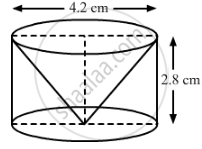# From a solid cylinder of height 2.8 cm and diameter 4.2 cm, a conical cavity of the same height and same diameter is hollowed out. Find the total surface area of the remaining solid [take π=22/7] - Mathematics

From a solid cylinder of height 2.8 cm and diameter 4.2 cm, a conical cavity of the same height and same diameter is hollowed out. Find the total surface area of the remaining solid [take π=22/7]

#### Solution

The following figure shows the required cylinder and the conical cavity.Given:
Height (h) of the conical part = Height (h) of the cylindrical part = 2.8 cm
Diameter of the cylindrical part = Diameter of the conical part = 4.2 cm
∴ Radius (r) of the cylindrical part = Radius (r) of the conical part = 2.1 cm
Slant height (l) of the conical part =sqrt(r^2+h^2)

=sqrt((2.1)^2+(2.8)^2)cm

=sqrt(4.41+7.84)cm

=sqrt(12.25)cm=3.5 cm

Total surface area of the remaining solid = Curved surface area of the cylindrical part + Curved surface area of the conical part + Area of the cylindrical base

=2pirh+pirl+pir^2

=(2xx22/7xx2.1xx2.8+22/7xx2.1xx3.5+22/7xx2.1xx2.1) cm^2

=(36.96+23.1+13.86)cm^2

=73.92 cm^2

Thus, the total surface area of the remaining solid is 73.92 cm2.

Concept: Surface Area of a Combination of Solids
Is there an error in this question or solution?
Chapter 14: Surface Areas and Volumes - Exercise 14.2 [Page 62]

#### APPEARS IN

RD Sharma Class 10 Maths
Chapter 14 Surface Areas and Volumes
Exercise 14.2 | Q 30 | Page 62

Share